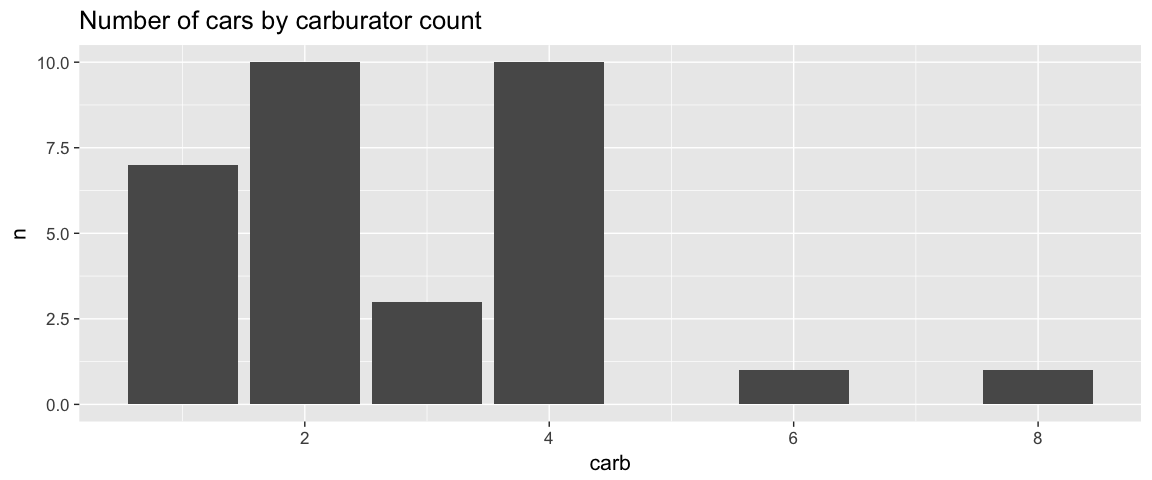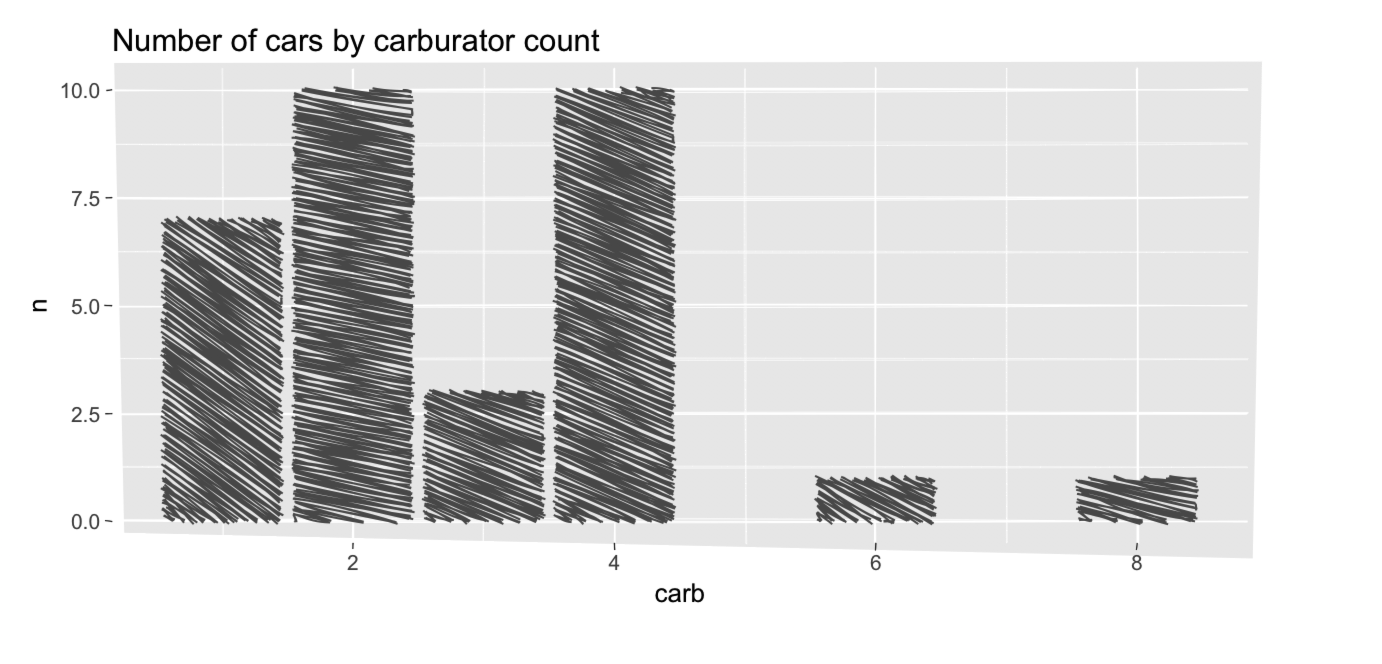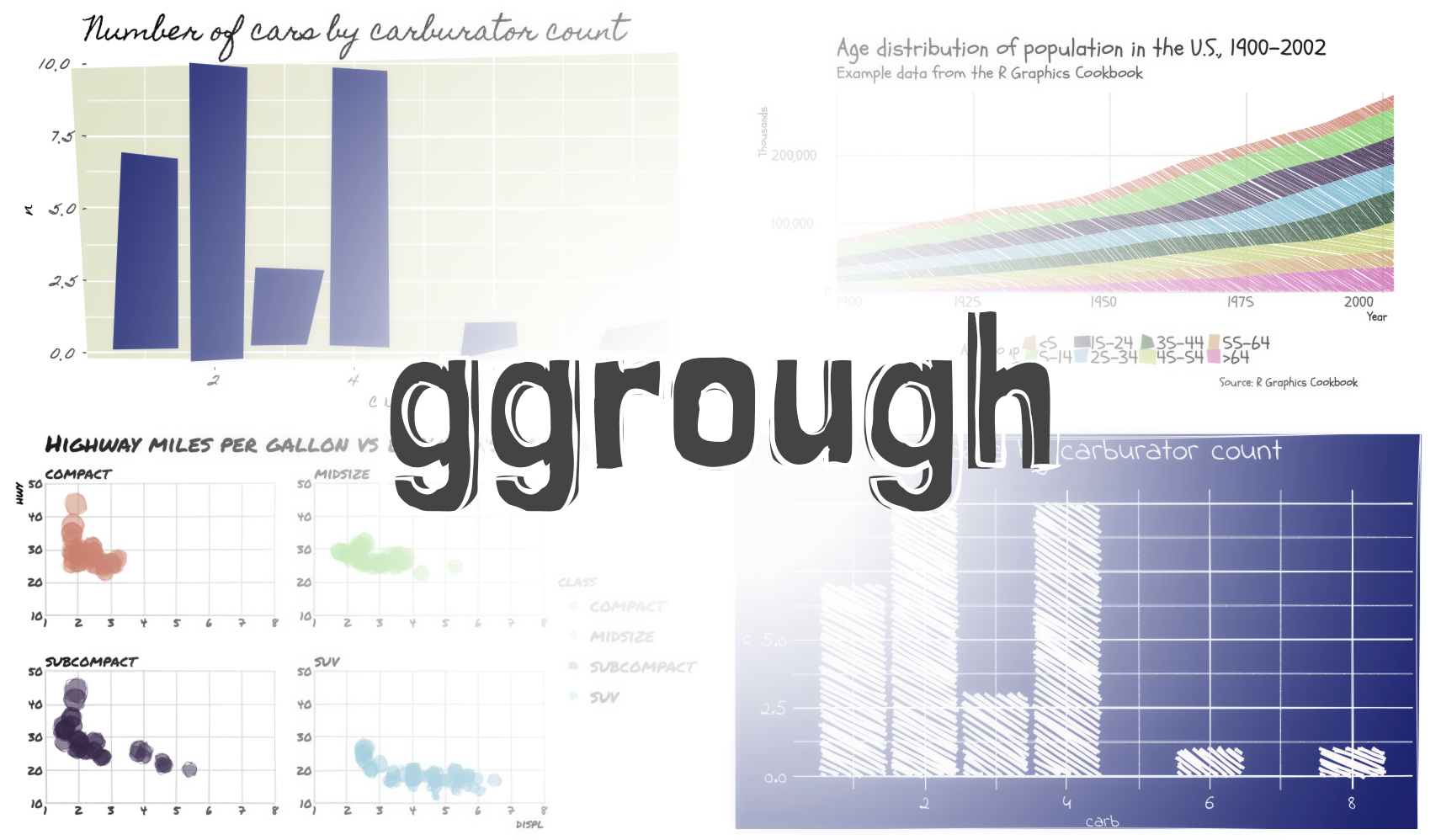``````require(dplyr)
library(ggplot2)
count(mtcars, carb) %>%
ggplot(aes(carb, n)) +
geom_col() +
labs(title="Number of cars by carburator count") +
theme_grey(base_size = 16) -> p
p
````````````library(ggrough)
options <- list(
Background=list(roughness=8),
GeomCol=list(fill_style="zigzag", angle_noise=0.5, fill_weight=2))
get_rough_chart(p, options)
``````